Get instant live expert help with Excel or Google Sheets“My Excelchat expert helped me in less than 20 minutes, saving me what would have been 5 hours of work!”

#### Post your problem and you’ll get Expert help in seconds.

Your message must be at least 40 characters
Our professional Expert are available now. Your privacy is guaranteed.

# Add decimal hours to time

Excel has a unique way of storing time through numbers, making it possible to perform mathematical operations to values of time.  This step by step tutorial will assist all levels of Excel users in adding decimal hours to time by using two methods.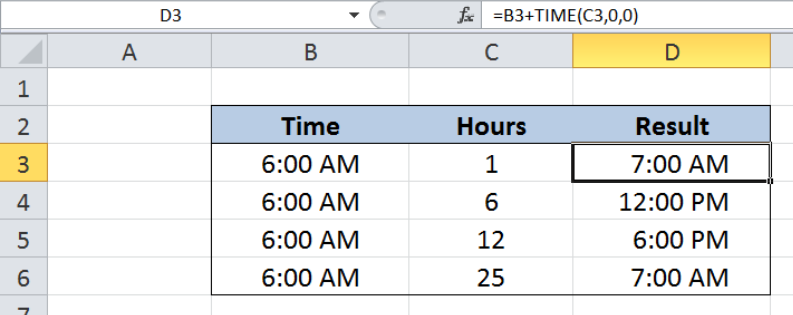Figure 1.  Final result: Add decimal hours to time

Formula 1: `=B3+TIME(C3,0,0)`

Formula 2: `=B3+C3/24`

## Syntax of TIME Function

TIME function is a built-in function in Excel that returns the decimal value of a particular time.  It is very useful when adding time because it allows compartmentalization of time into hours, minutes and seconds.

`=TIME(hour, minute, second)`

• When using this formula, we need to enter the time in this specific order : hours, minutes and then seconds.

## Setting up our Data

Our table contains three columns: Time (column B), Hours (column C) and Result (column D).  We want to add the number of hours in column C to the time in column B. The results will be recorded in column D.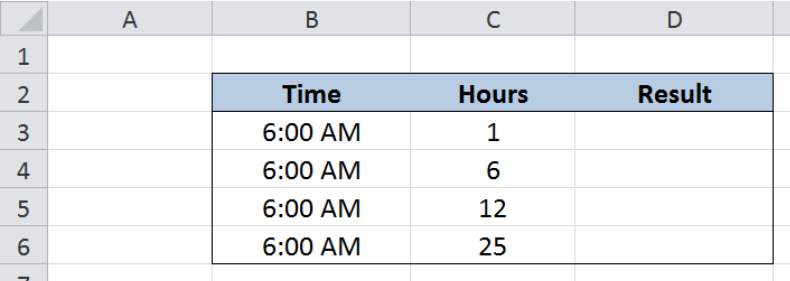Figure 2.  Sample data to add decimal hours to time

There are two ways to add decimal hours to time:

• By using the TIME function
• By adding the number of hours that is divided by 24

## Adding hours using TIME function

We want to add the hours in column C to the start time in column B by using the TIME function.  Let us follow these steps:

Step 1.  Select cell D3

Step 2.  Enter the formula: `=B3+TIME(C3,0,0)`

Step 3.  Press ENTER

Step 4.  Copy the formula in D3 to cells D4:D6 by clicking the “+” icon at the bottom-right corner of cell D3 and dragging it down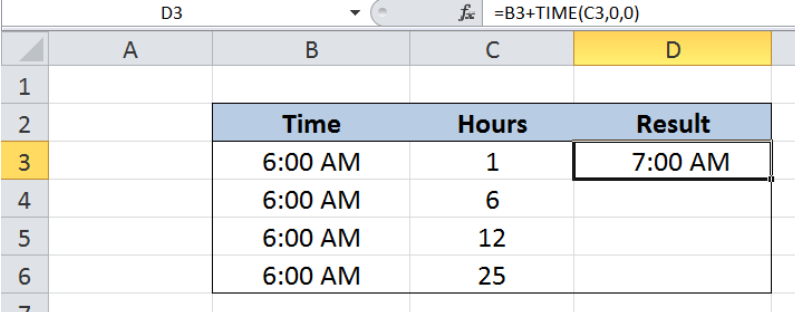Figure 3.  Adding decimal hours to time using the TIME function

In cell D3, 1 decimal hour is represented by the TIME function as TIME(1,0,0). Our formula then adds one decimal hour to 6:00 AM.  As a result, the time in D3 is 7:00 AM.

Below table shows the resulting time by using TIME function for D3 to D6.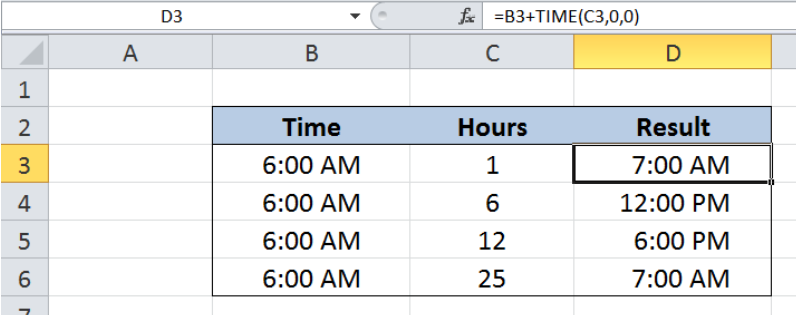Figure 4.  Output: Add decimal hours to time using TIME function

The use of TIME function is very straightforward because we input the number of hours, minutes and seconds separately, which is less prone to errors.  However, the TIME function rolls over back to zero in excess of 24 hours. Hence, if we input 25 hours to the TIME function, it will only be equivalent to 1 decimal hour.

## Adding hours divided by 24

Excel uniquely stores times as numbers, where the value for one whole day is 1.  There are 24 hours in one day. Hence, one hour is stored as a fraction of one day or 1/24.

Another way of adding decimal hours to time is by dividing the number of hours by 24.  Let us follow these steps:

Step 1.  Select cell D3

Step 2.  Enter the formula: `=B3+C3/24`

Step 3.  Press ENTER

Step 4.  Copy the formula in D3 to cells D4:D6 by clicking the “+” icon at the bottom-right corner of cell D3 and dragging it down

In cell D3, we divide the number of hours in C3 by 24, then add it to the time in B3.  Our formula adds one decimal hour to 6:00 AM. The resulting time in D3 is 7:00 AM, which is the same as in our previous example.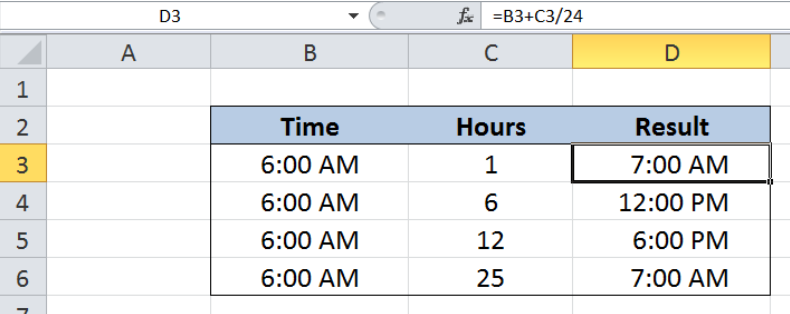Figure 5.  Output: Add hours divided by 24 to time

## Subtracting decimal hours to time

When we want to perform the opposite and subtract decimal hours to time, we just have to change the mathematical operation from addition to subtraction.  However, negative values for time results to error values in Excel.

The work-around is to use the following formula:

`=MOD(time-(hours/24),1)`

The MOD function ensures that the result has the same sign as the divisor.  In this formula, the divisor is 1, which is a positive number. Hence, the result will always be a positive value.

Most of the time, the problem you will need to solve will be more complex than a simple application of a formula or function. If you want to save hours of research and frustration, try our live Excelchat service! Our Excel Experts are available 24/7 to answer any Excel question you may have. We guarantee a connection within 30 seconds and a customized solution within 20 minutes.

### Did this post not answer your question? Get a solution from connecting with the expert.Another blog reader asked this question today on Excelchat:
Solution examplesHello I have a list of codes, some of these a duplicated in one column. In another column I have a list of prices. I am trying to work out how I identify when the code and two prices match and when they are different please? thank you
Solved by O. C. in 40 minsI need to calculate the number of female board members and directors for each company, also the % of females and how they compare to number and % of men in the same firms. Only small firms should count and only board members and relevant associating functions.
Solved by K. D. in 41 minsI need to create a sheet like Column one # Column 2 Month Column 3 Date Column 4 Amount Column 5 Amount Column 6 is total of #4 n #5 Column 7 amount Column 8 is #5 reduce from # 7 any one can help me please
Solved by S. W. in 11 minsI'd like to create a set of random numbers that are selected based on an assigned probability. For example, if a biologist wants to visit two ends of a lake, one 80 percent of the time and the other 20 percent of the time but on random days,how do I create this schedule?
Solved by O. D. in 29 minscopying numbers from 1 column to another in filter mode brings irrelevant data over to new column
Solved by O. J. in 40 mins## Subscribe to Excelchat.coAnother blog reader asked this question today on Excelchat: